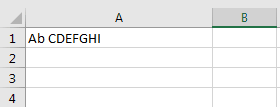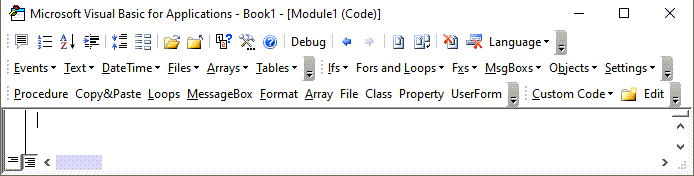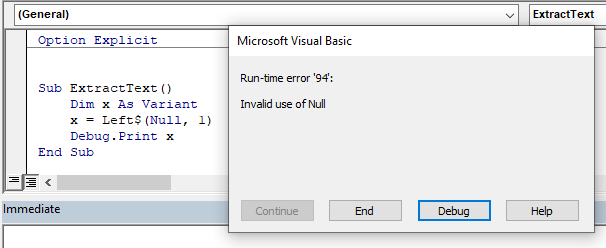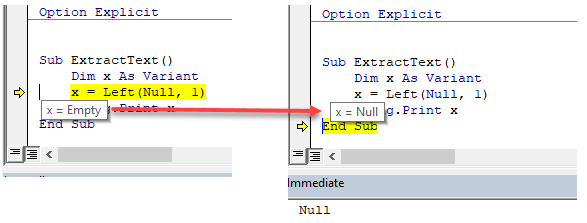# VBA Left (& Left\$) Function – Extract Text From Left

This tutorial will demonstrate how to use the Left VBA Function.

## Left Function

The VBA Left function returns the first n characters from a string.

### VBA Left Function n First Characters

The VBA Left function returns the first n characters from a string:

``````Sub LeftExample_1()
MsgBox Left("ABCDEFGHI", 4)        'Result is: "ABCD"
MsgBox Left("ABCDEFGHI", 2)        'Result is: "AB"
MsgBox Left("ABCDEFGHI", 1)        'Result is: "A"
MsgBox Left("ABCDEFGHI", 100)      'Result is: "ABCDEFGHI"
End Sub``````

### VBA Left Function n First Characters in a Variable

As shown above, you can define a string simply by entering text surrounded by quotation marks. But the LEFT Function will also work with string variables. These examples will extract the first n characters from a string variable.

``````Sub LeftExample_2()
Dim StrEx As String 'Define a string variable

StrEx = "ABCDEFGHI"

MsgBox Left(StrEx, 4)   'Result is: "ABCD"
MsgBox Left(StrEx, 2)   'Result is: "AB"
MsgBox Left(StrEx, 1)   'Result is: "A"
MsgBox Left(StrEx, 100) 'Result is: "ABCDEFGHI"

End Sub``````

### VBA Left Function n First Characters in a Cell

Strings can be defined in VBA code but also you can use values from cells. Read the value of a cell, keep it in a string variable, and extract n first characters from that Worksheet Cell value.``````Sub LeftExample_3()
Dim StrEx As String 'Define a string variable

'Read the value of cell A1 in worksheet Sheet1
StrEx = ThisWorkbook.Worksheets("Sheet1").Range("A1").Value

'For this example the value of cell A1 is "A bCDEFGHI"

MsgBox Left(StrEx, 4)   'Result is: "ABCD"
MsgBox Left(StrEx, 2)   'Result is: "AB"
MsgBox Left(StrEx, 1)   'Result is: "A"
MsgBox Left(StrEx, 100) 'Result is: "ABCDEFGHI"
End Sub``````

### VBA Left Function Trim off the Last Letter

To remove letters from the end of a string, use the LEFT Function along with the LEN Function.

The VBA LEN function counts the number of characters in a string:

``Len(StrEx)``

By combining the functions, we can remove a certain number of characters from the end of the string:

``````Sub LeftExample_4()
Dim StrEx As String 'Define a string variable

StrEx = "ABCDEF"

MsgBox Left(StrEx, Len(StrEx))     'Result is: "ABCDEF"
MsgBox Left(StrEx, Len(StrEx) - 1) 'Result is: "ABCDE"
MsgBox Left(StrEx, Len(StrEx) - 2) 'Result is: "ABCD"
End Sub``````

### VBA Left to Extract First Name

To extract the first name from a string with a full name, use the Left Function along with the Instr Function.

The VBA Instr function searches for a substring inside a string and returns the position number of the substring.

``InStr(StrEx, " ")``

By combining the functions, we can extract the first word from a phrase:

``````Sub LeftExample_5()
Dim StrEx As String 'Define a string variable

StrEx = "Alexander Graham Bell"

MsgBox Left(StrEx, InStr(StrEx, " "))
'Result is: "Alexander "                   (notice the space at the end)

MsgBox Left(StrEx, InStr(StrEx, " ") - 1)
'Result is: "Alexander"                   (NO space at the end)

StrEx = "Leonardo da Vinci"

MsgBox InStr(StrEx, " ")
'Result is: 9 because space is found in position 9

MsgBox Left(StrEx, InStr(StrEx, " ") - 1)
'Result is: "Leonardo"
End Sub``````

Stop searching for VBA code online. Learn more about AutoMacro - A VBA Code Builder that allows beginners to code procedures from scratch with minimal coding knowledge and with many time-saving features for all users!## VBA Left\$ Function vs Left Function

Both the Left and the Left\$ function will return the same answer if the variable where the data is being returned to is declared as a string. However, if the variable has been declared as a variant, then the Left function is allowed to return NULL values whereas the Left\$ function can only return string values.

If, for example, you are truing to return some data from an Access query, but the data returned is a NULL value, the Left\$ function will return an error.

Have a look at the code below:In the above example, even though our variable has been declared as a variant, the Left\$ function prevents the variable being populated with the NULL value.

In the code below however, the variable is populated with the NULL value.